• 2021-02-23 16:23:31

样本不平衡其实的主要思想就是过采样和欠采样，但是由于在复制少量标签的样本或者筛选大量标签的样本方法不同衍生出了不同的处理手段

1.随机过采样：复制标签少的样本使得好坏比满足建模需要

2.随机欠采样：抽取标签多的样本使得好坏比满足建模需要 （会丢失样本信息）

再进行标签多的样本欠采样，训练。

举个例子吧。假设你有1000个标签为1的样本，10个标签为0的样本。

第一步，从1样本中随机抽取10个和10个0样本组成训练样本

第二步，训练弱分类器发现，10个1样本中7个分类正确，3个分类错误。

第三步，将3个分类错误的1样本放回原来的990个1样本中。

重复第一步（这个时候抽样的1样本总样本量为993个）

5. KNN 欠抽样：虽然有很多变种，其实思想是大同小异的。

5.1 NM欠采样：有3个不同的方法

• NearMiss-1从多数类中选择，那些与少数类中三个最接近示例的平均距离最小的示例。
• NearMiss-2从多数类中选择，那些与从少数类中三个最远示例的平均距离最小的示例。
• NearMiss-3涉及为少数类中最接近的每个示例，选择给定数量的多数类示例。

举个栗子，假设你有1000个标签为1的样本，10个标签为0的样本

NearMiss-1就是每个1样本去找到离他最近的3个0样本（欧氏距离），计算平均距离。然后选取平均距离小的n个1样本（满足建模的好坏样本比就可以）

NearMiss-2就是每个1样本去找到离他最远的3个0样本，计算平均距离。选取平均距离最小的n个1样本

NearMiss-3就是考虑每个0样本，去找到距离0样本最近的n个样本，组成训练样本

可以熟悉下下面这个库的使用

imblearn.under_sampling import NearMiss

5.2 浓缩最近邻（Condensed Nearest Neighbor（CNN））规则欠采样

栗子还是那个栗子

第一步，保留全部标签为0的样本

第二步，基于所有1样本和0样本对1样本进行knn（K=1）训练

第三步，保留分类错误的1样本

第四步，全部0样本和分类错误的1样本组成采样后的样本

这样会让0，1样本比较接近的样本放在一起，有利于进行边界决策，距离太远就不用考虑啦，要是能训练出区分这些比较接近的样本的模型才是重点。

5.3 Tomek链接的欠采样

CNN欠采样的变种，目的是清除边界上的样本，一些分布非常相似但是标签模棱两可的样本

Tomek链接定义：

（i）实例a的最近邻居是b，（ii）实例b的最近邻居是a，并且（iii）实例a和b属于不同的类，则实例a和b定义Tomek链接

将有Tomek链接的样本删除组成训练样本

Tomek的目的是剔除边界上的样本，但是并不是一个特别好用的欠采样技术，一般是结合其他欠采样方法如CNN使用（OSS单面选择欠采样）

5.4 编辑最近邻（Edited Nearest Neighbors （ENN））规则欠采样

也是一个脑洞非常神奇的采样设计，目的是剔除边界样本

先用K=3对1样本进行分类，将分类错误的1样本剔除，再结合CNN（K=1）采样

5.5 欠采样的邻居清洁规则（NCR）

其实就是先用CNN再用ENN

总结一下

KNN进行欠采样其实关注的就是两个点

1. 清除离边界很远的冗余样本

2.清除离边界很近的模糊样本

6. SMOTE算法过采样

不复杂，对于0样本找到最近的0样本，或者随机最近的n个0样本中的一个

然后对于两个0样本x差值中间随机选一个值生成新的0样本

比如 0样本1的 x1 = 1 ， 0样本1的x1 = 4

那么生成的0样本 x1 = (4-1) * rand(0,1) + 1

保证生成的0样本的x取值在两个样本之间就可以了

7.Borderline-SMOTE算法

SMOTE算法的一种优化，对于哪些0样本选取作为基准来进行生成新的0样本

如果说0样本周围k个样本中50%以上都是1样本，而且不是100%都是1样本（如果100%的话会被认为是噪声），就选取这个0样本进行SMOTE插值

8. KMeans过采样

第一步，对1样本和0样本分别进行KMeans聚类

第二步，对于样本少的类别进行过采样（解决内部不平衡问题）

第三步，对于1样本和0样本进行过采样（解决样本标签不平衡问题）数据挖掘
更多相关内容
• ## 正负样本不平衡处理方法总结

万次阅读 多人点赞 2017-12-29 12:08:53
1， Bootstrapping，hard negative mining 最原始的一种方法，主要使用在传统的机器学习方法中。 比如，训练随机森林，...比如，SVM分类中去掉那些离分界线较远的样本，只保留离分界线较近的样本。 2， heurist...

1， Bootstrapping，hard negative mining
最原始的一种方法，主要使用在传统的机器学习方法中。
比如，训练随机森林，对于每一个树就是采样booststraping方法采样，也算是随机森林的其中一个随机性表现。再比如bagging方法，也是基于该有放回重采样方法。

比如，SVM分类中去掉那些离分界线较远的样本，只保留离分界线较近的样本。

2， heuristic sampling

标准的faster rcnn中，假设正样本IOU(0.7~1.0)。负样本IOU(0.0~0.3)。比如实际的RPN网络中，实际最后的anchor经过NMS处理后的负样本是很多的，假如有30000个。这里面只有少数的正样本，大部分都是负样本。在RPN模块中，仅仅对其中大约1/3的hard的proposal进行了2分类和回归的loss计算。即只从3000个proposal里面只选择那些hard负样本，这样实际训练出来的效果最好。但是后续的roi pooling部分，只需要传递256个proposal进行计算既可。即正的proposal128个，负的proposal128个。假设正的不够，则使用负的进行补齐。

3， online hard example mining(OHEM)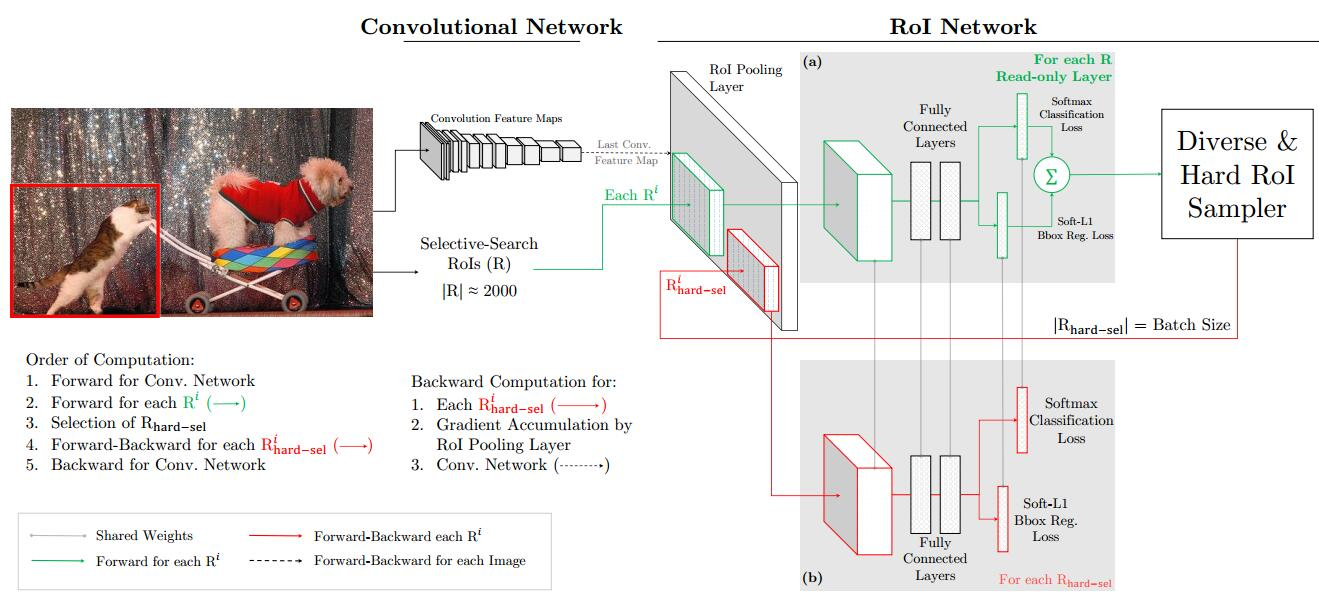其中，一个trick就是，在绿色的网络进行前向传播完，其中出来的好多ROI会存在一些Loss较高，但是这些ROI有很大的IOU的情况，这样就会使得梯度重复计算和传播，因此，这里，作者加入了NMS进行IOU的过滤。

4，Focal Loss
出自Focal Loss for Dense Object Detection这篇文章，
文章重点就是提出了focal loss这个cross entropy (CE) loss的改进版，实现了对于正负样本不平衡的调整。具体思路就是其公式，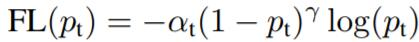从这个公式就可以分析出，
假设r=2，pt分数为0.9，那么这个easy example的loss将会被缩小0.01a倍
假设r=2，pt分数为0.968，那么这个easy example的loss将会被缩小0.001a倍
假设r=2，pt分数为0.1，那么这个hard example的loss将会被缩小0.81a倍
同样所有样本的loss都会缩小，但是hard example要不easy example缩小的小，从而取得好的训练效果。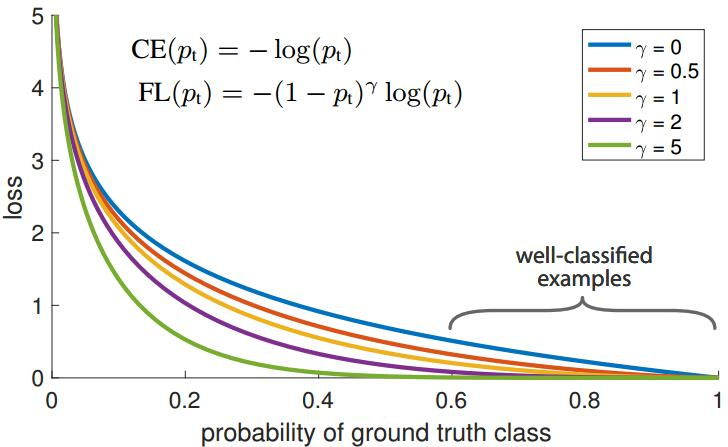从上图也可以反映出，r>0的曲线的loss要比r=0的曲线的更低，loss更小。

当然文章还提出了一个RetinaNet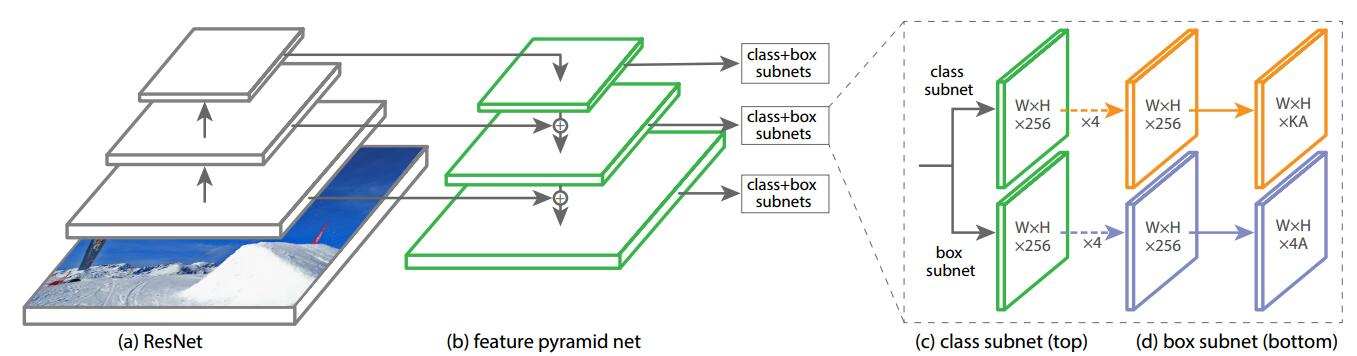RetinaNet以Resnet为基础结构，通过Feature Pyramid Network (FPN)产生多尺度的输出特征图，然后分别级联一个分类和回归的子网络。这点和faster RCNN有点区别，在faster中是只使用一个网络进行分类和回归操作，RetinaNet将2个任务分离后，也许会在精度上有一定提高吧，也更容易训练。
这里一个trick是RetinaNet的初始化，
(1)除了分类子网络的最后一层，其余层w全部初始化为u=0, σ = 0:01的高斯分布,b初始化为0。
(2)最后一个分类的卷积层，b初始化为- log((1 - π)/π)，文中使用π = 0.01，这样初始化使得每个anchor被标记为前景的概率为0.01，
这里b的这个公式是怎么得出的呢？
最终的分类得分是一个逻辑回归，公式为,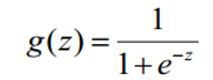这里的z=wx+b，由于w初始化为u=0, σ = 0:01的高斯分布，所以，z=b,最终的概率设为π，从而得出公式，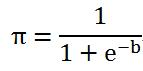从而解出，b=- log((1 -π)/π)

这个初始化对于focal loss的训练很重要。

5，class balanced cross-entropy

出自Holistically-nested edge detection这篇文章， 主要用于FCN,U-net等分割，边缘检测的网络，用于对像素级别的2分类样本不平衡进行优化。

sigmoid_cross_entropy公式:

-y_hat* log(sigmoid(y)) - (1 - y_hat) * log(1 - sigmoid(y))

class_balanced_sigmoid_cross_entropy公式:

-β*y_hat* log(sigmoid(y)) -(1-β) * (1 - y_hat) * log(1 - sigmoid(y))

思想就是引入新的权值β，实现正负样本loss的平衡，从而实现对不同正负样本的平衡。

6, local rank，PISA，ISR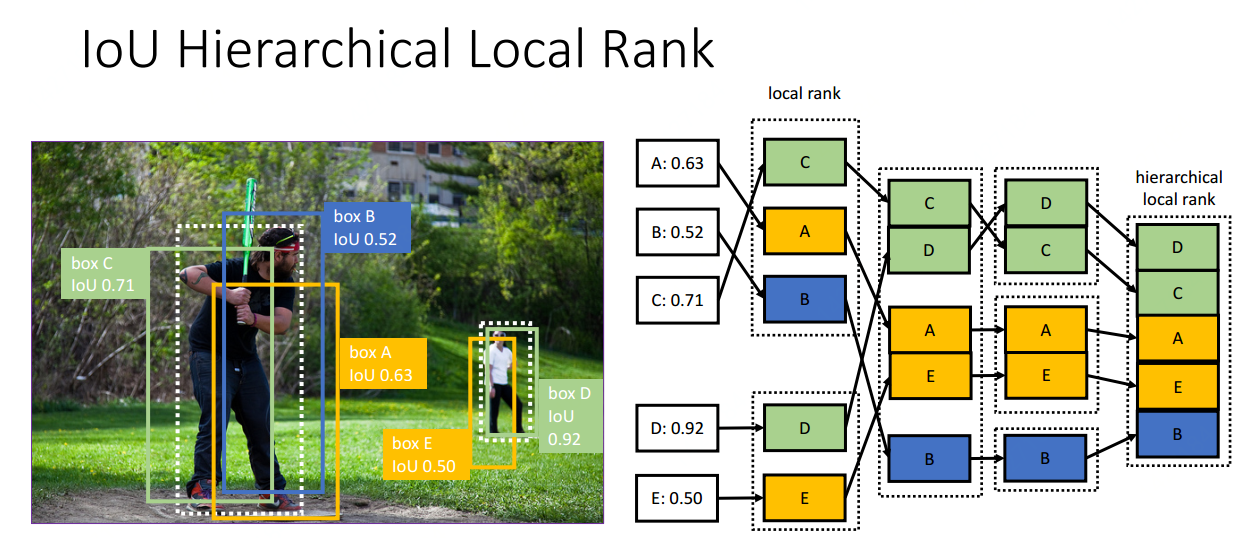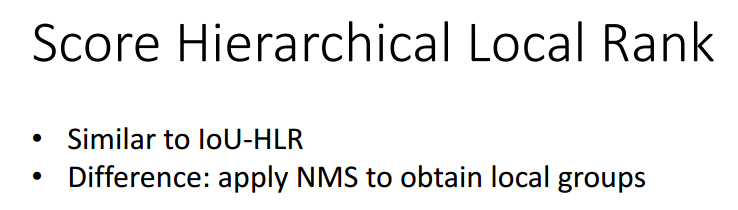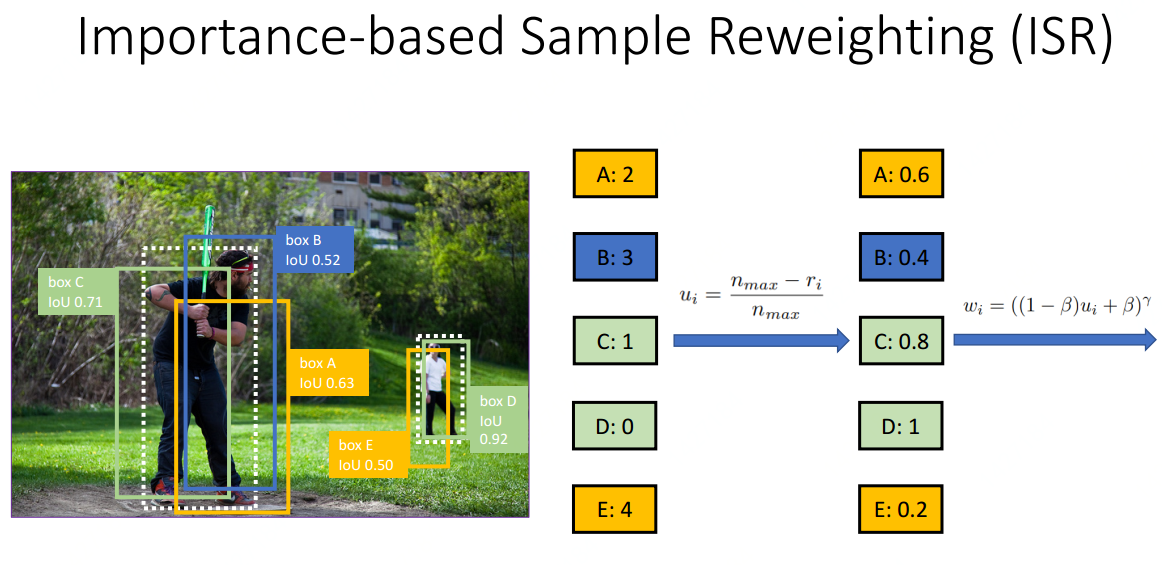References：

https://github.com/abhi2610/ohem

Libra R-CNN: Towards balanced learning for object detection

展开全文• 在分类中如何处理训练集中不平衡问题   在很多机器学习任务中，训练集中可能会存在某个或某些类别下的样本数远大于另一些类别下的样本数目。即类别不平衡，为了使得学习达到更好的效果，因此需要解决该类别不平衡...

### 在分类中如何处理训练集中不平衡问题

在很多机器学习任务中，训练集中可能会存在某个或某些类别下的样本数远大于另一些类别下的样本数目。即类别不平衡，为了使得学习达到更好的效果，因此需要解决该类别不平衡问题。

### Jason Brownlee 的回复：

原文标题：8 Tactics to Combat Imbalanced Classes in Your Machine Learning Dataset
你在对一个类别不均衡的数据集进行分类时得到了90%的准确度（Accuracy）。当你进一步分析发现，数据集的90%的样本是属于同一个类，并且分类器将所有的样本都分类为该类。在这种情况下，显然该分类器是无效的。并且这种无效是由于训练集中类别不均衡而导致的。
首先举几个所收到的邮件中关于类别不均衡的例子：

• 在一个二分类问题中，训练集中class 1的样本数比class 2的样本数是60:1。使用逻辑回归进行分类，最后结果是其忽略了class 2，即其将所有的训练样本都分类为class 1。
• 在分类任务的数据集中，有三个类别，分别为A，B，C。在训练集中，A类的样本占70%，B类的样本占25%，C类的样本占5%。最后我的分类器对类A的样本过拟合了，而对其它两个类别的样本欠拟合。

### 什么是类别不均衡问题

类别数据不均衡是分类任务中一个典型的存在的问题。简而言之，即数据集中，每个类别下的样本数目相差很大。例如，在一个二分类问题中，共有100个样本（100行数据，每一行数据为一个样本的表征），其中80个样本属于class 1，其余的20个样本属于class 2，class 1:class2=80:20=4:1，这便属于类别不均衡。当然，类别不均衡问同样会发生在多分类任务中。它们的解决方法是一样的。因此，为了便于讨论与理解，我们从二分类任务入手进行讲解。

### 类别不均衡问题是现实中很常见的问题

大部分分类任务中，各类别下的数据个数基本上不可能完全相等，但是一点点差异是不会产生任何影响与问题的。
在现实中有很多类别不均衡问题，它是常见的，并且也是合理的，符合人们期望的。如，在欺诈交易识别中，属于欺诈交易的应该是很少部分，即绝大部分交易是正常的，只有极少部分的交易属于欺诈交易。这就是一个正常的类别不均衡问题。又如，在客户流失的数据集中，绝大部分的客户是会继续享受其服务的（非流失对象），只有极少数部分的客户不会再继续享受其服务（流失对象）。一般而已，如果类别不平衡比例超过4:1，那么其分类器会大大地因为数据不平衡性而无法满足分类要求的。因此在构建分类模型之前，需要对分类不均衡性问题进行处理。
在前面，我们使用准确度这个指标来评价分类质量，可以看出，在类别不均衡时，准确度这个评价指标并不能work。因为分类器将所有的样本都分类到大类下面时，该指标值仍然会很高。即，该分类器偏向了大类这个类别的数据。

### 八大解决方法

• 扩大数据集
当遇到类别不均衡问题时，首先应该想到，是否可能再增加数据（一定要有小类样本数据），更多的数据往往战胜更好的算法。因为机器学习是使用现有的数据多整个数据的分布进行估计，因此更多的数据往往能够得到更多的分布信息，以及更好分布估计。即使再增加小类样本数据时，又增加了大类样本数据，也可以使用放弃一部分大类数据（即对大类数据进行欠采样）来解决。
• 尝试其它评价指标
从前面的分析可以看出，准确度这个评价指标在类别不均衡的分类任务中并不能work，甚至进行误导（分类器不work，但是从这个指标来看，该分类器有着很好的评价指标得分）。因此在类别不均衡分类任务中，需要使用更有说服力的评价指标来对分类器进行评价。如何对不同的问题选择有效的评价指标参见这里
上面的超链接中的文章，讲述了如何对乳腺癌患者复发类别不均衡数据进行分类。在文中，推荐了几个比传统的准确度更有效的评价指标：

• 混淆矩阵(Confusion Matrix)：使用一个表格对分类器所预测的类别与其真实的类别的样本统计，分别为：TP、FN、FP与TN。

• 精确度(Precision)
• 召回率(Recall)
• F1得分(F1 Score)：精确度与找召回率的加权平均。

特别是：

• Kappa (Cohen kappa)
• ROC曲线(ROC Curves):见Assessing and Comparing Classifier Performance with ROC Curves

• 对数据集进行重采样
可以使用一些策略该减轻数据的不平衡程度。该策略便是采样(sampling)，主要有两种采样方法来降低数据的不平衡性。

• 对小类的数据样本进行采样来增加小类的数据样本个数，即过采样（over-sampling ，采样的个数大于该类样本的个数）。

• 对大类的数据样本进行采样来减少该类数据样本的个数，即欠采样（under-sampling，采样的次数少于该类样本的个素）。

采样算法往往很容易实现，并且其运行速度快，并且效果也不错。更详细的内容参见这里

一些经验法则：

• 考虑对大类下的样本（超过1万、十万甚至更多）进行欠采样，即删除部分样本；
• 考虑对小类下的样本（不足1为甚至更少）进行过采样，即添加部分样本的副本；
• 考虑尝试随机采样与非随机采样两种采样方法；
• 考虑对各类别尝试不同的采样比例，比一定是1:1，有时候1:1反而不好，因为与现实情况相差甚远；
• 考虑同时使用过采样与欠采样。

• 尝试产生人工数据样本
一种简单的人工样本数据产生的方法便是，对该类下的所有样本每个属性特征的取值空间中随机选取一个组成新的样本，即属性值随机采样。你可以使用基于经验对属性值进行随机采样而构造新的人工样本，或者使用类似朴素贝叶斯方法假设各属性之间互相独立进行采样，这样便可得到更多的数据，但是无法保证属性之前的线性关系（如果本身是存在的）。
有一个系统的构造人工数据样本的方法SMOTE(Synthetic Minority Over-sampling Technique)。SMOTE是一种过采样算法，它构造新的小类样本而不是产生小类中已有的样本的副本，即该算法构造的数据是新样本，原数据集中不存在的。该基于距离度量选择小类别下两个或者更多的相似样本，然后选择其中一个样本，并随机选择一定数量的邻居样本对选择的那个样本的一个属性增加噪声，每次处理一个属性。这样就构造了更多的新生数据。

• 尝试不同的分类算法
强烈建议不要对待每一个分类都使用自己喜欢而熟悉的分类算法。应该使用不同的算法对其进行比较，因为不同的算法使用于不同的任务与数据。具体可以参见“Why you should be Spot-Checking Algorithms on your Machine Learning Problems”。
决策树往往在类别不均衡数据上表现不错。它使用基于类变量的划分规则去创建分类树，因此可以强制地将不同类别的样本分开。目前流行的决策树算法有：C4.5、C5.0、CART和Random Forest等。

• 尝试对模型进行惩罚

你可以使用相同的分类算法，但是使用一个不同的角度，比如你的分类任务是识别那些小类，那么可以对分类器的小类样本数据增加权值，降低大类样本的权值（这种方法其实是产生了新的数据分布，即产生了新的数据集，译者注），从而使得分类器将重点集中在小类样本身上。一个具体做法就是，在训练分类器时，若分类器将小类样本分错时额外增加分类器一个小类样本分错代价，这个额外的代价可以使得分类器更加“关心”小类样本。如penalized-SVM和penalized-LDA算法。

Weka中有一个惩罚模型的通用框架CostSensitiveClassifier，它能够对任何分类器进行封装，并且使用一个自定义的惩罚矩阵对分错的样本进行惩罚。
如果你锁定一个具体的算法时，并且无法通过使用重采样来解决不均衡性问题而得到较差的分类结果。这样你便可以使用惩罚模型来解决不平衡性问题。但是，设置惩罚矩阵是一个复杂的事，因此你需要根据你的任务尝试不同的惩罚矩阵，并选取一个较好的惩罚矩阵。

• 尝试一个新的角度理解问题
我们可以从不同于分类的角度去解决数据不均衡性问题，我们可以把那些小类的样本作为异常点(outliers)，因此该问题便转化为异常点检测(anomaly detection)与变化趋势检测问题(change detection)。

异常点检测即是对那些罕见事件进行识别。如通过机器的部件的振动识别机器故障，又如通过系统调用序列识别恶意程序。这些事件相对于正常情况是很少见的。
变化趋势检测类似于异常点检测，不同在于其通过检测不寻常的变化趋势来识别。如通过观察用户模式或银行交易来检测用户行为的不寻常改变。
将小类样本作为异常点这种思维的转变，可以帮助考虑新的方法去分离或分类样本。这两种方法从不同的角度去思考，让你尝试新的方法去解决问题。

• 尝试创新
仔细对你的问题进行分析与挖掘，是否可以将你的问题划分成多个更小的问题，而这些小问题更容易解决。你可以从这篇文章In classification, how do you handle an unbalanced training set?中得到灵感。例如：

• 将你的大类压缩成小类；
• 使用One Class分类器（将小类作为异常点）；
• 使用集成方式，训练多个分类器，然后联合这些分类器进行分类；
….

这些想法只是冰山一角，你可以想到更多的有趣的和有创意的想法去解决问题。更多的想法参加Reddit的文章http://www.quora.com/In-classification-how-do-you-handle-an-unbalanced-training-set

### 选择某一种方法并使用它

你不必成为一个精通所有算法的算法奇才或者一个建立准确而可靠的处理数据不平衡的模型的统计学家，你只需要根据你的问题的实际情况从上述算法或方法中去选择一种或两种方法去使用。希望上述的某些方法能够解决你的问题。例如使用其它评价指标或重采样算法速度快并且有效。

### 总结

记住，其实并不知道哪种方法最适合你的任务与数据，你可以使用一些启发式规则或经验去选择某一个较优算法。当然最好的方法测试每一种算法，然后选择最好的方法。最重要的是，从点滴开始做起，根据自己现有的知识，并不断学习去一步步完善。

这里有一些我认为有价值的可供参考的相关资料，让你进一步去认识与研究数据不平衡问题：

相关书籍
Imbalanced Learning: Foundations, Algorithms, and Applications
相关论文
Data Mining for Imbalanced Datasets: An Overview
Learning from Imbalanced Data
Addressing the Curse of Imbalanced Training Sets: One-Sided Selection (PDF)
A Study of the Behavior of Several Methods for Balancing Machine Learning Training Data

### Sergey Feldman的回答：

• 将大类中样本划分到L个聚类中，然后训练L个分类器，每个分类器使用大类中的一个簇与所有的小类样本进行训练得到。最后对这L个分类器采取少数服从多数对未知类别数据进行分类，如果是连续值（预测），那么采用平均值。

• 设小类中有N个样本。将大类聚类成N个簇，然后使用每个簇的中心组成大类中的N个样本，加上小类中所有的样本进行训练。
• 无论你使用前面的何种方法，都对某个或某些类进行了损害。为了不进行损害，那么可以使用全部的训练集采用多种分类方法分别建立分类器而得到多个分类器，采用投票的方式对未知类别的数据进行分类，如果是连续值（预测），那么采用平均值。

• 在最近的ICML论文中，表明增加数据量使得已知分布的训练集的误差增加了，即破坏了原有训练集的分布，从而可以提高分类器的性能。这篇论文与类别不平衡问题不相关，因为它隐式地使用数学方式增加数据而使得数据集大小不变。但是，我认为破坏原有的分布是有益的。

• More details than you need: imho, the most interesting of the corrupting distributions is the blankout distribution, where you just zero out a random subset of features. Why is it interesting? Because you are helping your classifier be sturdier/hardier by giving it variations of your data that have essentially missing features. So it has to learn to classify correctly even in adverse conditions. 一个相关的想法是，在神经网络中，随机选择部分隐藏层单元来继续训练（即，随机去掉一部分隐藏层单元，(zeroed-out)）。具体见http://web.stanford.edu/~sidaw/cgi-bin/home/lib/exe/fetch.php?media=papers:fastdropout.pdf

### Kripa Chettiar的回答：

• 增加新数据，可以使用SMOTE或SMOTEBoost产生人造数据。
• 将大类压缩。压缩比例需要具体情况具体分析，取决于你所拥有的数据。例如，A类中有30个样本，B类中有4000个样本，那么你可以将B类压缩成1000（进行采样）。
• 可以结合1与2
• 对于那种极小类是异常点的分类任务，因此分类器需要学习到大类的决策分界面，即分类器是一个单个类分类器（One Class Classifier）。Weka中有相关的库。

### Dan Levin的回答：

一个很好的方法去处理非平衡数据问题，并且在理论上证明了。这个方法便是由Robert E. Schapire于1990年在Machine Learning提出的”The strength of weak learnability” ，该方法是一个boosting算法，它递归地训练三个弱学习器，然后将这三个弱学习器结合起形成一个强的学习器。我们可以使用这个算法的第一步去解决数据不平衡问题。
首先使用原始数据集训练第一个学习器L1。
然后使用50%在L1学习正确和50%学习错误的的那些样本训练得到学习器L2，即从L1中学习错误的样本集与学习正确的样本集中，循环一边采样一个。
接着，使用L1与L2不一致的那些样本去训练得到学习器L3。
最后，使用投票方式作为最后输出。
那么如何使用该算法来解决类别不平衡问题呢？
假设是一个二分类问题，大部分的样本都是true类。让L1输出始终为true。使用50%在L1分类正确的与50%分类错误的样本训练得到L2，即从L1中学习错误的样本集与学习正确的样本集中，循环一边采样一个。因此，L2的训练样本是平衡的。L使用L1与L2分类不一致的那些样本训练得到L3，即在L2中分类为false的那些样本。最后，结合这三个分类器，采用投票的方式来决定分类结果，因此只有当L2与L3都分类为false时，最终结果才为false，否则true。
自己已经在实践中使用过很多次，并且效果都不错。

### Kaushik Kasi的回答：

• 对小类中的样本进行复制以增加该类中的样本数，但是可能会增加bias。
• 对小类中的样本通过调整特征值来人工生成样本，而使得该类中样本个数增多。如在图像中，对一幅图像进行扭曲得到另一幅图像，即改变了原图像的某些特征值。但是该方法可能会产生现实中并存在的样本。

### Muktabh Mayank的回答：

这里有一个类似SVM的方法来处理不平衡问题。具体参见这里。

展开全文机器学习
• 计算机视觉中的性能最好的目标检测方法主要分为两种: one-stage 和two-stage 方法。two-stage方法分为两步，第一步为候选区域生成阶段(Proposal stage),通过如Selective Search、EdgeBoxes等方法可以生成数量相对较...

# 1. 前言

目前。计算机视觉中的性能最好的目标检测方法主要分为两种: one-stage 和two-stage 方法。two-stage方法分为两步，第一步为候选区域生成阶段(Proposal stage),通过如Selective Search、EdgeBoxes等方法可以生成数量相对较小候选目标检测框；第二步为分类与回归阶段，对第一阶段生成的 Candiate Proposal 进行分类和位置回归。one-stage 代表性的方法是R-CNN系列，如 R-CNN， Fast R-CNN, Faster R-CNN。 而one-stage 方法直接对图像的大量 Candiate Proposals 进行分类与回归。

这两类方法中均存在类别不平衡问题，two-stage 方法通过第一步已经将当量的候选区域降低到一个数量较小的范围，同时又在第二步通过一些启发式原则，将正负样本的比例降低到一定程度。而 one-stage 优于没有这降低候选框的步骤，因此，候选区域的数量大大超过 two-stage 方法，因此，在精度上，two-stage 仍然优于 one-stage 方法，但是在速度和模型复杂度上， one-stage 占优势。

类别不平衡会使检测器评估 1 0 4 − 1 0 6 10^4-10^6 的候选位置，但是通常只有少量的位置存在目标，这回导致两个问题:

1. 由于大量的 easy negative 对模型的训练没有提供很大的作用，因此训练很低效；
2. 由于在训练中 easy negatives 在数据中占主导，因此这些样本会覆盖少量的正样本的损失（即负样本的损失梯度占主导），从而导致模型退化。

因此，解决样本不平衡问题是提高目标检测精度的一个关键技术之一。

# 2. OHEM

OHEM 是通过改进 Hard Example Mining 方法，使其适应online learning算法特别是基于SGD的神经网络方法。Hard Example Mining 通过交替地用当前样本集训练模型，然后将模型固定，选择 False Positive 样本来重新组建用于下一次模型训练的样本集。但是因为训练神经网络本事就是一个耗时的操作，这样在训练中固定模型，这样会急剧降低模型的训练进程。

Hard Examples Mining通常有两种方法：

1. 用于优化 SVM 的方法： 该方法首先需要一个工作的样本集，用来训练 SVM， 使其在该样本集上收敛，然后通过某些原则来添加或删除某些样本以更新该样本集。该原则是：删除那些简单样本(Easy Examples), 即分类正确的概率高与某个阈值(个人理解，原话The rule removes examples that are “easy” in the sense that they are correctly classified beyond the current model’s margin. Conversely)， 添加那些困难那样本，即分类正确的概率低于某个阈值(the rule adds new examples that are hard in the sense that they violate the current model’s margin)

2. 用于非SVM的方法：该方法首先用完整的数据集中的正样本和一个随机的负样本及来构成开始的训练样本集，然后训练模型使其在该训练集上收敛，然后完整的数据集中选择 False Positive 添加到训练样本集中。

OHEM算法的大致流程是: 首先计算出每个ROI的loss， 然后按loss从高到低来排列每个 ROI， 然后为每张图片选择 B / N B/N 个损失最高的 ROI 作为Hard Examples，其中 B 表示总的 ROI 数量， N N 表示batch-size 的大小，在 Fast R-CNN 中， N=2， B=128时，效果很好。

但是如果直接按照 loss 对所有的 ROI 进行选择，会有一个缺点，由于 ROI 很多，这样 很多 ROI 的位置就会相关并重叠，如果和某个高 Loss 的 ROI 重合度很高的其它 ROI很多， 这样， 这些 ROI 的 Loss 通常也会很多，这样这些样本都会被选择，但是它们可以近似认为时同一个，这样就会给其它较低 Loss 的 ROI 更少的选择余地，这样就会存在冗余。为了消除这种冗余，作者提出先使用 NMS (non-maximum suppression) 删除部分重合度很高的 ROI， 在使用上述方法进行 选择 Hard Example。

实现技巧:

论文，作者将该方法是现在 Fsat R-CNN 目标检测方法中。最简单做法是更改损失函数层，损失函数层首先计算所有 ROI 的 loss， 然后根据 loss 对 ROI 进行排序，并选择 hard RoIs， 让 那些 non-RoIs的损失变为0. 这种方法虽然很简单，但是非常不高效，因为还需要为所有的 RoIs 分配进行反向传播时需要的内存空间。

为了克服这个缺点，作者对下面的 Figure 1 进行改进， 如下面的 Figure 2.该改进时使用两份同样的 RoI network。 其中一个是只读的(readonly), 即只进行前向计算，不进行反向传播优化，所以只需要为前向传播分配内存，它的参数实时保持和另一个 RoI network（regular RoI network)保持一样。在每次迭代时，首先使用 readonly RoI network 对每个 ROI 计算起 loss，然后用上面描述的选择 hard RoIs 的方法选择 hard RoIs. 然后利用 regular RoI network来对选择的 hard RoIs 进行前向和后向计算来优化网络。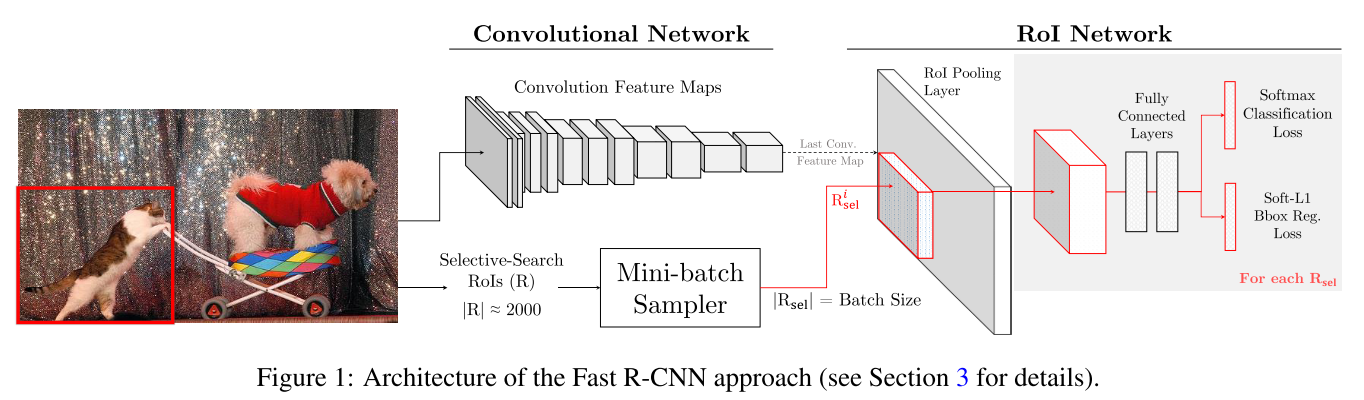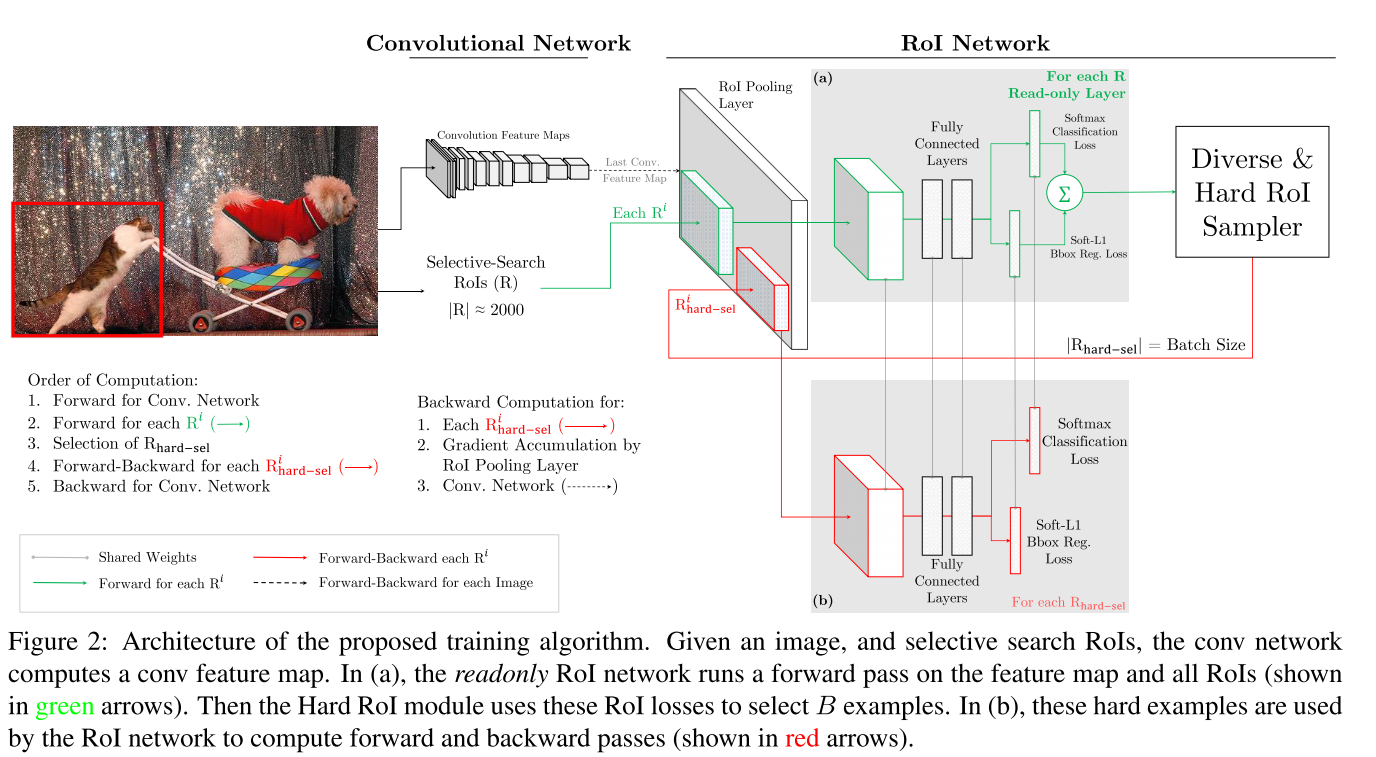# 3. Focal Loss

## 3.1 Cross Entropy

在改论文中，作者认为样本类别的不平衡可以归结为难易样本的不平衡，从而更改交叉熵损失函数，重新对样本赋予不同的权值，之前的模型这些样本都是同等重要的，从而使模型训练更加关注 hard examples。

首先引入交叉熵的公式:

(公式1) C E ( p , y ) = { − log ⁡ ( p ) , i f y = = 1 − log ⁡ ( 1 − p ) , o t h e r w i s e CE(p, y)=\begin{cases} -\log (p), \quad if \quad y == 1 \\ -\log(1-p), \quad otherwise \end{cases} \tag{公式1}

其中， y ∈ { − 1 , 1 } y\in\{-1, 1\} ,表示真实类别， p ∈ [ 0 , 1 ] p\in[0,1] 表示我们预测的概率，为了方便，我们定义:

(公式2) p t = { p , i f y = = 1 1 − p , o t h e r w i s e p_t=\begin{cases} p, \quad if \quad y == 1 \\ 1-p, \quad otherwise \end{cases} \tag{公式2}

因此， C E ( p , y ) = C E ( p t ) = − log ⁡ ( p t ) CE(p,y)=CE(p_t)=-\log(p_t) ,该方法在 p t p_t 较大时，该loss是一个较小的量级， 如下图的连线所示所示，因为存在大量的易分类样本，相加后会淹没正样本的loss。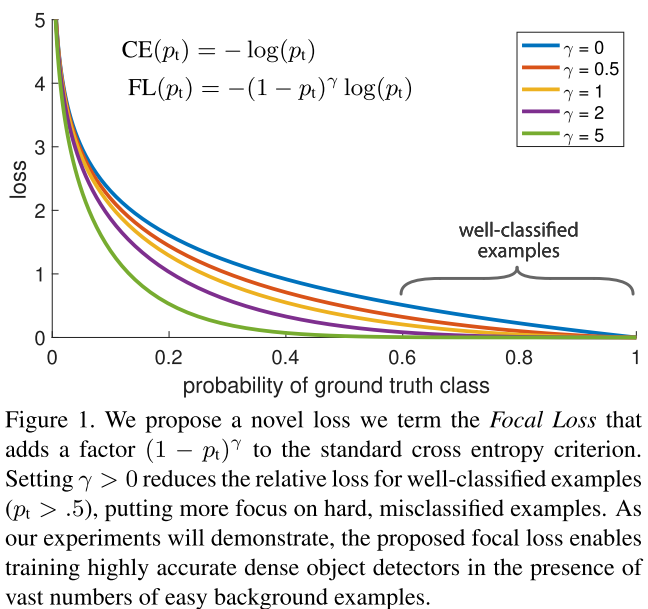## 3.2 Balanced Cross Entropy

一个常见的解决类别不平衡的方式是引入一个加权因子 α ∈ [ 0 , 1 ] \alpha \in [0,1] 来表示正样本的权重， 1 − α 1-\alpha 表示负样本的权重。我们按照定义 p t p_t 的方法重新定义 α \alpha α t \alpha_t , α − b a l a n c e C E l o s s \alpha-balance\quad CE \quad loss 定义如下:

(公式3) C E ( p , y ) = C E ( p t ) = − α t log ⁡ ( p t ) CE(p,y)=CE(p_t)=-\alpha_t\log(p_t) \tag{公式3}

## 3.3 Focal Loss

α − b a l a n c e C E l o s s \alpha-balance\quad CE \quad loss 虽然可以平衡 positive和negative的重要性，但是对 easy/hard 样本还是无法区分， Focal loss 通过更 Cross loss来达到区分easy/hard的目的:

(公式4) F L ( p t ) = − ( 1 − p t ) γ log ⁡ ( p t ) FL(p_t)=-(1-p_t)^\gamma\log(p_t) \tag{公式4}

上图展示了不同 γ \gamma 取值对应的 loss，通过分析上述公式，我们发现，当 p t p_t 非常小时，即样本被分类错误，此时 ( 1 − p t ) γ (1-p_t)^\gamma 接近1， loss几乎不受影响，当 p t p_t 接近于1时，即样本被分类正确，此时 ( 1 − p t ) γ (1-p_t)^\gamma 接近0，此时降低了该样本的权重，比如，取 γ = 2 \gamma=2 , 当时 p t = = 0.9 p_t==0.9 时，该样本的 loss 会降低100倍，

在实际应用中，作者使用了该 Focal loss的变体，即加入了 α \alpha 平衡因子:

(公式5) F L ( p t ) = − α t ( 1 − p t ) γ log ⁡ ( p t ) FL(p_t)=-\alpha_t(1-p_t)^\gamma\log(p_t) \tag{公式5}

## 3.4 模型初始化

作者提出，对于二分类，一般模型的模型初始化会同概率的对待正负样本，由于类别不平衡，负样本的 loss 会占主导，作者提出在训练初始阶段对正样本的概率估计“prior”的概念，用 π \pi 表示， 通过设置它来达到正样本的输出概率低的效果，比如为0.01，从而使模型更加关注正样本。实际操作中，出了最后一个用于分类的卷积层，其余卷积层的参数初始化为bias b = 0 b=0 ，而最后一层 b = − log ⁡ ( ( 1 − π ) / π ) b=-\log((1-\pi)/\pi) , 实验中设置为 0.01.

两个实现细节

1. 计算 total loss 时，是对一张图片上所有的 ~100k 的anchors 的 focal loss求和，而并不像使用启发式的 RPN 和 OHEM 使用部分小的anchors来计算 loss。 然后通过拥有 gt box 的anchors的数量来进行归一化，不适用所有 anchors 的数量进行归一化的原因是: 由于anchors 存在大量的 easy examples， 因此得到的 Focal loss非常小，如果使用所有 anchors 的数量进行归一化，回导致归一化后的 loss 非常小。

2. 当只使用 α \alpha 时， 将 α \alpha 偏向样本少的类别，当同时使用 α 和 γ \alpha 和 \gamma 时，他们需要向相反方向变化，论文中设置为 α = 0.25 ， γ = 2 \alpha=0.25，\gamma=2 .

改论文提出 Focal Loss 存在两个缺点:

1. loss 存在两个超参数，调节该参数是一个费力的操作；
2. 该 loss 是一个不会随着数据分布变化而变化的静态 loss。

该论文有一句概括该篇论文的核心思想的一句话: 类别的不平衡可以归结为难易样本的不平衡，难易样本的不平衡可以归结为梯度的不平衡原话如下:

In this work, we first point out that the class imbalance can be summarized to the imbalance in difficulty and the imbalance in difficulty can be summarized to the imbalance in gradient norm distribution.

如下图所示: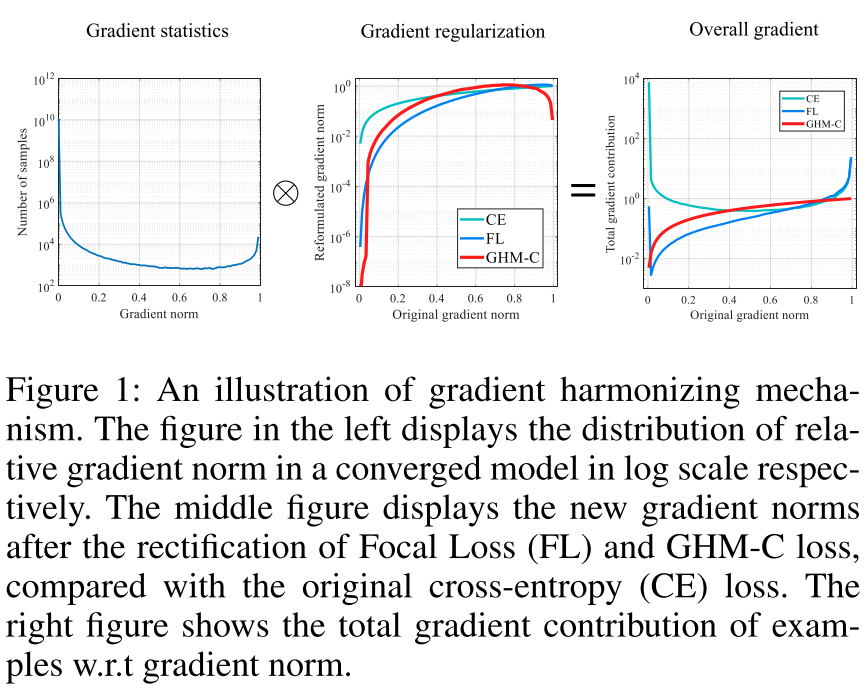左边是样本数量关于梯度的分布，中间表示各个修正后的函数的梯度（使用了log scale）对原始梯度的，右边表示所有样本集的梯度贡献的分布。

## 4.1 Cross Entropy

(公式6) L C E ( p , p ∗ ) = { − log ⁡ ( p ) , i f p ∗ = = 1 − log ⁡ ( 1 − p ) , i f p ∗ = = 0 L_{CE}(p, p^*)=\begin{cases} -\log (p), \quad if \quad p^* == 1 \\ -\log(1-p), \quad if \quad p^* == 0 \end{cases} \tag{公式6}

定义 x x 是模型未经过 sigmoid 之前的输出， 则 p = s i g m o i d ( x ) p = sigmoid(x) ,得出该损失函数对 x x 的梯度为:

(公式7) ∂ L C E ( p , p ∗ ) ∂ x = { p − 1 , i f p ∗ = = 1 p , i f p ∗ = = 0 = p − p ∗ \begin{aligned} \frac{\partial L_{CE}(p, p^*)}{\partial x} &amp;=\begin{cases} p - 1, \quad if \quad p^* == 1 \\ p, \quad if \quad p^* == 0 \end{cases} \\ &amp;= p - p^* \end{aligned} \tag{公式7}

定义梯度的模长(norm) g g 为：
(公式6) g = ∣ p − p ∗ ∣ = { 1 − p , i f p ∗ = = 1 p , i f p ∗ = = 0 g=|p-p^*|=\begin{cases} 1-p, \quad if \quad p^* == 1 \\ p, \quad if \quad p^* == 0 \end{cases} \tag{公式6}

(公式7) G D ( g ) = 1 l ϵ ∑ k = 1 N δ ϵ ( g k , g ) GD(g)=\frac{1}{l_\epsilon}\sum_{k=1}^{N}\delta_{\epsilon}(g_k, g) \tag{公式7}

其中， g k g_k 是第 k 个样本的gradient norm，

(公式8) δ ϵ ( x , y ) = { 1 , i f y − ϵ 2 ≤ x &lt; y + ϵ 2 0 , i f o t h e r w i s e \delta_{\epsilon}(x, y)=\begin{cases} 1, \quad if \quad y-\frac{\epsilon}{2} \le x &lt; y + \frac{\epsilon}{2} \\ 0, \quad if \quad otherwise \end{cases} \tag{公式8}

(公式9) l ϵ ( g ) = m i n ( g + ϵ 2 , 1 ) − m a x ( g − ϵ 2 , 0 ) l_{\epsilon}(g) = min(g+\frac{\epsilon}{2}, 1) - max(g-\frac{\epsilon}{2}, 0) \tag{公式9}

这个公式可以理解为，以梯度 g g 为中心，宽度为 ϵ \epsilon 的区域内的样本密度。

梯度密度协调参数:

(公式10) β i = N G D ( g i ) = 1 G D ( g 1 ) / N \beta_i=\frac{N}{GD(g_i)} = \frac{1}{GD(g_1)/N} \tag{公式10}

分母是对梯度位于 g i g_i 范围的部分样本进行归一化，如果所有样本的梯度时均分分布，那么对于任意 g i g_i 都有 G D ( g i ) = N GD(g_i)=N .(这里不是很理解为什么N，可以理解它们相等)

通过将梯度密度协调参数将 GHM 嵌入到损失函数中，则 GHM-C Loss 为:

(公式11) L G H M − C = 1 N ∑ i = 1 N β i L C E ( p i , p i ∗ ) = ∑ i = 1 N L C E ( p i , p i ∗ ) G D ( g i ) \begin{aligned} L_{GHM-C}&amp;=\frac{1}{N}\sum_{i=1}^{N}\beta_iL_{CE}(p_i, p_i^*) \\ &amp;=\sum_{i=1}^{N}\frac{L_{CE}(p_i, p_i^*)}{GD(g_i)} \end{aligned} \tag{公式11}

## 4.3 Unit Region Approximation

计算公式11时，求和有一个N，再求 G D ( g i ) GD(g_i) 时会遍历所有的样本，因此该公式的时间复杂度为 O ( N 2 ) O(N^2) .如果并行的化，每个计算单元也有N的计算量。对gradient norm进行排序的最好的算法复杂度为 O ( N log ⁡ N ) O(N\log N) ，然后用一个队列去扫描样本得到梯度密度的时间复杂度为 n 。基于排序的方法即使并行也不能较快的计算，因为N往往是 1 0 5 10^5 甚至 1 0 6 10^6 ，仍然是非常耗时的.

作者提出的近似求解的方法如下:

1. 将 g 划分为 M = 1 ϵ M=\frac{1}{\epsilon} 个单元
2. r j = [ ( j − 1 ) ϵ , j ϵ ] r_j=[(j-1)\epsilon, j\epsilon] 表示第 j 个单元， R j R_j 表示 r j r_j 中的样本数量， i n d ( g ) = t ind(g)=t 表示 g g 属于第几个 unit。

根据上述定义，得出近似梯度密度函数为:

(公式12) G D ^ ( g ) = R i n d ( g ) ϵ = i n d ( g ) m \hat {GD}(g)=\frac{R _{ind(g)}}{\epsilon}=ind(g)m \tag{公式12}

(公式13) β i ^ = N G D ^ ( g i ) \hat {\beta_i}=\frac{N}{\hat {GD}(g_i)} \tag{公式13}

(公式14) L ^ G H M − C = 1 N ∑ i = 1 N β ^ i L C E ( p i , p i ∗ ) = ∑ i = 1 N L C E ( p i , p i ∗ ) G D ^ ( g i ) \begin{aligned} \hat L_{GHM-C}&amp;=\frac{1}{N}\sum_{i=1}^{N}\hat \beta_iL_{CE}(p_i, p_i^*) \\ &amp;=\sum_{i=1}^{N}\frac{L_{CE}(p_i, p_i^*)}{\hat {GD}(g_i)} \end{aligned} \tag{公式14}

利用上面的公式，由于我们可以事先求好 β ^ \hat \beta , 在求和时只需查找 β ^ i \hat \beta_i 即可，因此时间复杂度为 O ( M N ) O(MN) .

因为loss的计算是基于梯度密度函数，而梯度密度函数根据一个batch中的数据得到，一个batch的统计结果是有噪声的。与batch normalization相同，作者用Exponential moving average来解决这个问题，也就是

(公式15) S j ( t ) = α S j ( t − 1 ) + ( 1 − α ) R J ( t ) G ^ D ( g ) = S i n d ( g ) ϵ = S i n d ( g ) M \begin{aligned} S_{j}^{(t)} &amp;= \alpha S_{j}^{(t-1)} + (1-\alpha) R_J^{(t)} \\ \hat GD(g) &amp;= \frac{S_{ind(g)}}{\epsilon} = S_{ind(g)}M \end{aligned} \tag{公式15}

## 4.4 GHM-R Loss

将模型鱼的的偏移量定义为 t = ( t x , t y , t w , t h ) t=(t_x, t_y, t_w, t_h) , 将真实的偏移量定义为 t ∗ = ( t x ∗ , t y ∗ , t w ∗ , t h ∗ ) t^*=(t_x^*, t_y^*, t_w^*, t_h^*) ,回归loss采用 Smooth L1 loss:

(公式16) L r e g = ∑ i ∈ x , y , w , h S L 1 ( t i − t i ∗ ) L_{reg}=\sum_{i\in{x, y, w, h}}SL_1(t_i-t_i^*) \tag{公式16}

其中
(公式17) S L 1 ( d ) = { d 2 2 δ i f ∣ d ∣ ≤ δ ∣ d ∣ − δ 2 o t h e r w i s e SL_1(d)=\begin{cases} \frac{d_2}{2\delta} \quad if |d|\le\delta \\ |d|-\frac{\delta}{2} \quad otherwise \end{cases} \tag{公式17}

L r e g L_{reg} 关于 d = t i − t i ∗ d=t_i-t_i^* 的梯度为:

(18) ∂ S L 1 ∂ t i = ∂ S L 1 ∂ d { d δ i f ∣ d ∣ ≤ δ s g n ( d ) o t h e r w i s e \frac{\partial SL_1}{\partial t_i}=\frac{\partial SL_1}{\partial d}\begin{cases} \frac{d}{\delta} \quad if |d| \le \delta \\ sgn(d) \quad otherwise \end{cases} \tag{18}

从公式可以看出，当样本操作 ∣ d ∣ |d| 时， 所有样本都有相同的梯度 1， 这就使依赖梯度范数来区分不同样本是不可能的， 一种简单的替代方法时直接使用 ∣ d ∣ |d| 作为衡量标准，但是该值理论上无限大，导致 U n i t R e g i o n A p p r o x i m a t i o n Unit Region Approximation 无法实现，

为了将 GHM 应用到回归损失上，作者修改了原始的 S L 1 SL_1 损失函数:

(19) A S L 1 ( d ) = d 2 + u 2 − u ASL_1(d) = \sqrt{d_2+u^2}-u \tag{19}

该函数和 S L 1 SL_1 具有类似的属性，当d的绝对值很小时，近似 L2 loss， 当d的绝对值比较大时， 近似 L1 loss， A S L 1 ASL_1 关于d的梯度为:

(20) ∂ A S L 1 ∂ d = d d 2 + u 2 \frac{\partial ASL_1}{\partial d} = \frac{d}{\sqrt{d^2+u^2}} \tag{20}

这样就将梯度值限制在 [ 0 , 1 ) [0,1)

定义 d r = ∣ d d 2 + u 2 ∣ dr=|\frac{d}{\sqrt{d^2+u^2}}| , 则 GHM-R Loss 为:

(21) L G H M − R = 1 N ∑ i = 1 N β i A S L 1 ( d i ) = ∑ i = 1 N A S L 1 ( d i ) G D ( g r i ) \begin{aligned} L_{GHM-R}&amp;=\frac{1}{N}\sum_{i=1}^N\beta_i ASL_1(d_i) \\ &amp;=\sum_{i=1}^N\frac{ASL_1(d_i)}{GD(gr_i)} \end{aligned} \tag{21}

# 5.PISA

PISA 方法和 Focal loss 和 GHM 有着不同， Focal loss 和 GHM 是利用 loss 来度量样本的难以程度，而本篇论文作者从 mAP 出法来度量样本的难易程度。

作者提出提出改论文的方法考虑了两个方面:

1. 样本之间不应该是相互独立的或同等对待。基于区域的目标检测是从大量候选框中选取一小部分边界框，以覆盖图像中的所有目标。因此，不同样本的选择是相互竞争的，而不是独立的。一般来说，检测器更可取的做法是在确保所有感兴趣的目标都被充分覆盖时，在每个目标周围的边界框产生高分，而不是对所有正样本产生高分。作者研究表明关注那些与gt目标有最高IOU的样本是实现这一目标的有效方法。

2. 目标的分类和定位是有联系的。准确定位目标周围的样本非常重要，这一观察具有深刻的意义，即目标的分类和定位密切相关。具体地，定位好的样本需要具有高置信度好的分类。

## 5.1 Prime Samples

Prime Samples 是指那些对检测性能有着巨大影响的样本。作者研究表明样本的重要程度依赖于它和ground truth 的 IoU值，因此作者提出了一种 IOU-HLR 排序。

在目标检测中时如何定义正样本（True Positive)的呢？

1. Bouding box 和他最近的 GT 的IoU 大于一个阈值 θ \theta
2. 如果该 GT 没有大于阈值的 Bouding Box，则选择最大的 IoU。

剩余的标注为负样本。

mAP 的原理揭露了对目标检测器更重要的两个准则:

1. 在所有和gt目标重合的边界框中，IoU最高的边界框时最重要的，因为它的IoU值直接影响召回率。

2. 所有不同目标的最高IoU边界框中,具有更高的IoU的边界框更加重要,因为它是随着 θ \theta 增加最后一个低于阈值 θ \theta 的边界框,从而对整体精度有很大的影响。

## 5.2 IoU Hierarchical Local Rank (IoU-HLR)

基于上述分析，作者提出了一种称为 IoU-HLR 的排序方法，它既反映了局部的IoU关系(每个ground truth目标周围)，也反映了全局的IoU关系(覆盖整个图像或小批图像)。值得注意的是，不同于回归前的边界框坐标，IoU-HLR是根据样本的最终定位位置来计算的，因为mAP是根据回归后的样本位置来计算的。

该排序方法的大致流程如下图所示，其原理如下:

1. 首先将所有的样本根据理他最近的 GT ，将他们分到不同的组；
2. 然后在每个组内，按照 IoU对它们进行降序排列，我们得到 IoU Local Rank（IoU-LR);
3. 我们对 IoU-LR,进行采样，即将每个组内的TOP1的样本采样出来放在一个组，以此类推对 TOP2, TOP3，一次进行；
4. 最后对上面采样的样本，在每个组内进行降序排序，就得到最终的 IoU-HLR.

IoU-HLR遵循上述两个准则。首先，它通过局部排序（即上面的步骤2）将每个单独的 GT 的 对应的样本中 IoU 较高的样本放在前面，其次通过重采样和排序(步骤3， 4)将不同 GT 的 对应的样本中， 将 IoU 较高的放在了前面。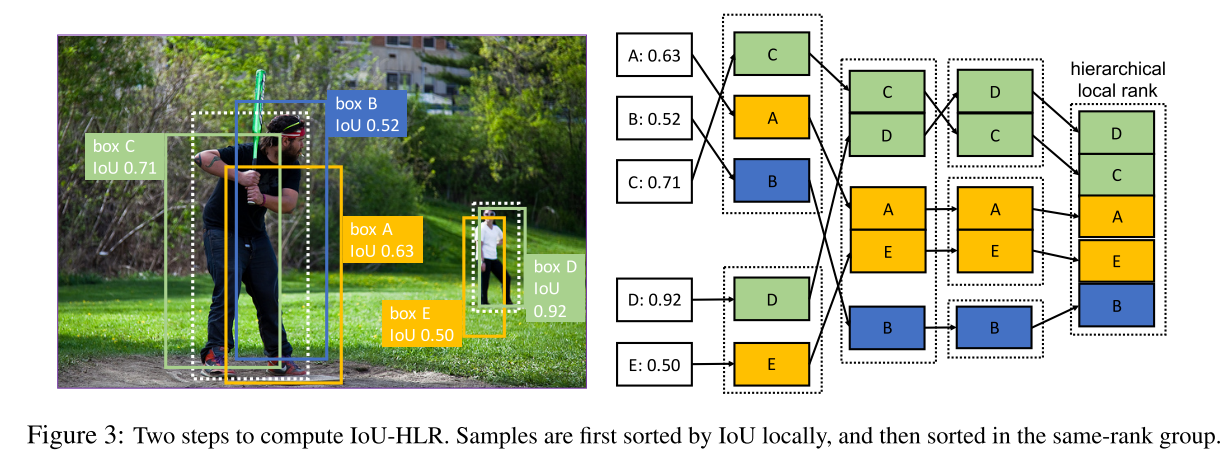## 5.3 Learn Detectors via Prime Sample Attention

作者提出Prime Sample Attention，一种简单且有效的采样策略，该采样策略将更多的注意力集中到 Prime examples 上， PISA 由两部分组成: Importance- based Sample Reweighting(ISR)和Classification Aware Regression Loss(为CARL).

PISA 的训练过程是基于 prime samples 而不是同等对待所有样本。

1. 首先 prime samples 的权重要大于其他样本的权重， 所以分类往往在这些样本上预测更高的分数；
2. 用一个联合目标函数来对分类器和回归其进行学习，因此prime sample的分数相对于不重要样本的分数将会得到提高。

### 5.3.1 Importance-based Sample Reweighting

作者提出一种基于 soft sampling 的方法: Importance-based Sample Reweighting (ISR), 他给不同样本根据重要性赋予不同的权重。首先它将Iou-HLR排序转化为线性映射的真实值。 IoU-HLR在每个类中分别进行计算。对于类 j j , 假设总共有 n j n_j 个样本， 通过 IoU-HLR 表示为 { r 1 , r 2 , ⋯ &ThinSpace; , r n j } \{r_1,r_2,\cdots,r_{n_j} \} . 其中 0 ≤ r i ≤ n j − 1 0 \le r_i \le n_j -1 ,使用一个线性转换函数将 r i r_i 转换为 u i u_i , 表示第 j j 类中的第 i i 个样本的重要程度:
(22) u i = n j − r i n j u_i = \frac{n_j-r_i}{n_j} \tag{22}

采用指数函数的形式来京一部将样本重要性 u i u_i 转换为 loss 的权值 w i w_i , γ \gamma 表示对重要样本给予多大的优先权的程度因子， β \beta 决定最小样本权值的偏差(感觉就是一个决定最小的权值大小的一个变量)。

(23) w i = ( ( 1 − β ) u i + β ) γ w_i=((1-\beta)u_i + \beta)^\gamma \tag{23}

根据上面得到的权重值，重写交叉熵:

(24) L c l s = ∑ i = 1 n w i ′ C E ( s i , s i ∗ ) + ∑ i = n + 1 m C E ( s i , s i ∗ ) w i ′ = w i ∑ i = 1 n C E ( s i , s i ∗ ) ∑ i = 1 n w i C E ( s i , s i ∗ ) \begin{aligned} L_{cls} &amp;= \sum_{i=1}^{n}w_i^{&#x27;}CE(s_i, s_i^*) + \sum_{i=n+1}^{m}CE(s_i, s_i^*) \\ w_i^{&#x27;} &amp;= w_i \frac{\sum_{i=1}^{n}CE(s_i, s_i^*)}{\sum_{i=1}^{n}w_iCE(s_i, s_i^*)} \end{aligned} \tag{24}

其中 n 和 m 分别表示真样本和负样本的数量， s i s_i s i ∗ s_i^* 分别表示预测分数和分类目标，需要注意的是，如果只是简单的添加 loss 权值将会改变 loss 的值，并改变正负样本的比例，因此为了保持正样本的总的 loss 值不变， 作者将 w i w_i 归一化为 w i ′ w_i^{&#x27;}
(这里不是很理解，欢迎大家解惑)

### 5.3.2 Classification-Aware Regression Loss

5.3.1 已经介绍如何染个分类器知道 prime samples， 那么如何让回归其也知道 prime sample，作者提出了 Classification-Aware Regression Loss（CARL) 来联合优化分类器和回归其两个分支。CARL可以提升主要样本的分数，同时抑制其他样本的分数。回归质量决定了样本的重要性，我们期望分类器对重要样本输出更高的分数。两个分支的优化应该是相互关联的，而不是相互独立的。

作者的方法是让回归器知道分类器的分数，这样梯度就可以从回归器传播到分期其分支。公式如下:

(25) L r e g = ∑ i = 1 n c i ′ L ( d i , d ^ i ) c i ′ = c i ∑ i = 1 n L ( s i , s i ∗ ) ∑ i = 1 n c i L ( s i , s i ∗ ) c i = v i 1 n ∑ i = 1 n v i v i = ( ( 1 − b ) p i + b ) k \begin{aligned} L_{reg} &amp;=\sum_{i=1}^n c_i^{&#x27;}\mathcal{L}(d_i, \hat d_i) \\ c_i^{&#x27;} &amp;= c_i \frac{\sum_{i=1}^{n}\mathcal{L}(s_i, s_i^*)}{\sum_{i=1}^{n}c_i\mathcal{L}(s_i, s_i^*)} \\ c_i &amp;= \frac{v_i}{\frac{1}{n}\sum_{i=1}^{n}v_i} \\ v_i &amp;= ((1-b)p_i +b)^k \end{aligned} \tag{25}

p i p_i 表示相应类别的预测分数， d i d_i 表示输出的回归偏移量。利用一个指数函数将 p i p_i 转化为 v i v_i ，随后根据所有样本的平均值对它进行缩放。为了保持损失规模不变，对具有分类感知的 c i c_i 进行归一化。 L \mathcal{L} 是常用的smooth L1 loss。

L r e g L_{reg} 关于 c i ′ c_i^{&#x27;} 的梯度与原回归损失 L ( d i , d ^ i ) \mathcal{L}(d_i, \hat d_i) 成正比。 \$L_{reg} 关于 p i p_i 的梯度与 L ( d i , d ^ i ) \mathcal{L}(d_i, \hat d_i) 正相关。即回归损失越大的样本分类得分的梯度越大，说明对分类得分的抑制作用越强。从另一个角度看， L ( d i , d ^ i ) \mathcal{L}(d_i, \hat d_i) 反映了样本i的定位质量，因此可以认为是一个IoU的估计，进一步可以看作是一个IoU-HLR的估计。可以近似认为，排序靠前的样本有较低的回归损失，于是分类得分的梯度较小。对于CARL来说，分类分支受到回归损失的监督。 不重要样本的得分被极大的抑制掉，而对重要样本的关注得到加强。

待续。。。

# 7.总结

上面的方法大致可以分为两种：

1. Hard Sampling: 从所有样本中选择自己来训练模型: hard negative mining, OHEM
2. Soft sampling： 为样本赋予不同的权值: Focal Loss, GHM, PISA

Focal Loss认为正负样本的不平衡，本质上是因为难易样本的不平衡，于是通过修改交叉熵，使得训练过程更加关注那些困难样本，而GHM在Focal Loss的基础上继续研究，发现难易样本的不平衡本质上是因为梯度范数分布的不平衡，和Focal Loss的最大区别是GHM认为最困难的那些样本应当认为是异常样本，让检测器强行去拟合异常样本对训练过程是没有帮助的。PISA则是跳出了Focal Loss的思路，认为采样策略应当从mAP这个指标出发，通过IoU Hierarchical Local Rank (IoU-HLR)，对样本进行排序并权值重标定，从而使得recall和precision都能够提升。

展开全文• 文章目录数据不平衡为什么类别不平横会影响模型的输出？如何解决 数据不平衡 很多算法都有一个基本假设，那就是数据分布是均匀的。当我们把这些算法直接应用于实际数据时，大多数情况下都无法取得理想的结果。因为...SMOTE
• 为解决分类问题中效果受样本集类间平衡，并提高训练样本的多样性，可以使用K-Means欠采样对样本进行平衡处理。该方法利用K-means方法对大类样本聚类，形成与小类样本个数相同的簇类数，从每个簇中随机抽取单个样本...
• - 为什么样本不平衡时会出现问题 - 样本不平衡处理方式 - 样本不平衡时算法的评价指标
• 样本不平衡的影响2. 可尝试的改进方案2.1 采样2.2 数据增强2.3 loss2.4 模型层面2.5 评估指标 1. 样本不平衡的影响 ​ 举个例子，在广告CTR预估场景中，正负样本的占比可以达到1：500，点击的正样本是少数类，没...机器学习
• 对于分类算法，如果直接采用不平衡样本集进行训练学习，会存在一些问题。例如，如果正负样本比例达到1:99，则分类器简单地将所有样本都判定为负样本能达到99%的正确率，显然这并是我们想要的，我们想让分类器在...
• 在一个分类问题中，如果在所有你想要预测的类别里有一个或者多个类别的样本量非常少，那你的数据也许就面临不平衡类别的问题。 举例 1.欺诈预测（欺诈的数量远远小于真实交易的数量） 2.自然灾害预测（不好的事情远...
• 2 样本不平衡问题的处理方式2.1 场景2.2 对训练集还是测试集用？还是全量用？2.3 处理方法有哪些？ 待补充！ 2 样本不平衡问题的处理方式 2.1 场景 最近刚好做的项目是一个二分类问题，全量数据中正负样本比超过了5...Smote
• ## 样本不平衡处理

千次阅读 2019-05-27 17:28:15
采样最邻近算法，计算出每个少数类样本的K个近邻； 从K个近邻中随机挑选N个样本进行随机线性插值； 构造新的少数类样本； 将新样本与原数据合成，产生新的训练集； 代码实现： from imblearn.over_...
• 支持向量机(SVM)不平衡样本处理方法 在许多机器学习任务中经常出现样本不均衡问题，即某类样本在总样本占比极低。一般解决样本不平衡问题的方法有以下几种： （1）改变分类阈值，使分类结果更偏向于样本少的一类 （2...支持向量机 SVM
• 3.对于正负样本不均衡的解决方法 4.2中论文的翻译总结 一、 问题背分析 1.背景 在学术研究与教学中，很多算法都有一个基本假设，那就是数据分布是均匀的。当我们把这些算法直接应用于实际数据时，大多数情况下都...
• 分类时，由于训练集合中各样本数量均衡，导致模型训偏在...解决样本不均衡的方法主要包括两类：（1）数据层面，修改各类别的分布；（2）分类器层面，修改训练算法或目标函数进行改进。还有方法是将上述两类进行融合。
• 样本不平衡问题：数据集中，每个类别下的样本数目相差很大。以下以二分类问题为例进行讨论： 图示：坏样本占比：0.17%（ 492（坏样本） ： 284315（好样本）） 理想的样本应该是50：50，但...
• 当利用传统的分类算法处理不平衡数据时,由于多数类和少数类在数量上的倾斜,以总体分类精度最大为目标会使得分类模型偏向于多数类而忽略少数类,造成少数类的分类精度较低.如何针对不平衡数据分类问题设计分类算法,...
• 　搜集更多的数据来使数据达到平衡，但是一般出现样本不平衡问题，就是太可能以一种简单的方法获取到更多的样本（在金融领域，可以思考坏用户的定义是否过于严格） 1.2、数据增广： 目前数据增广主要应用于图像...
• 什么是样本不平衡？ 在计算机视觉（CV）任务里常常会碰到类别不平衡的问题， 例如： 图片分类任务：有的类别图片多，有的类别图片少 检测任务：现在的检测方法如SSD和RCNN系列，都使用anchor机制。 训练时正负...深度学习 神经网络 计算机视觉
• 无偏采样：意味着真实样本总体的类别比例在训练集中得以保持。 在训练集中正反例数目不同时，令表示正例数目，表示反例数目，观测几率为，，我们假设训练集是真是样本总体的无偏采样，因此...再缩放（再平衡）： ...
• 数据挖掘：数据清洗——数据不平衡处理 一、什么是数据平衡？ 平衡数据集指的是数据集各个类别的样本数目相差巨大，也叫数据倾斜。以二分类问题为例，即正类的样本数量远大于负类的样本数量。严格地讲，任何...
•研究论文
• 今天学习了关于样本类别分布均衡的处理的一些知识，在此和大家一起分享一下。 什么是样本类别分布均衡？ 举例说明，在一组样本中不同类别的样本量差异非常大，比如拥有1000条数据样本的数据集中，有一类样本的...
• ## 样本不平衡问题

千次阅读 2019-04-10 09:35:50
处理样本不平衡的原因： 一般而已，如果类别不平衡比例超过4:1，那么其分类器会大大地因为数据不平衡性而无法满足分类要求的。因此在构建分类模型之前，需要对分类均衡性问题进行处理。 在前面，我们使用准确度...机器学习
• 样本不均衡 样本不均衡指的是给定数据集中有的类别数据多，有的数据类别少，且数据占比较多的数据类别样本与占比较小的数据类别样本两者之间达到较大的比例。 常见解决办法 数据层面：采样，数据增强，数据合成等；...
• 当遇到不平衡数据时，以总体分类准确率为学习目标的传统分类算法会过多地关注多数类，从而使得少数类样本的分类性能下降。绝大多数常见的机器学习算法对于不平衡数据集都能很好地工作。 本文介绍几种有效的......